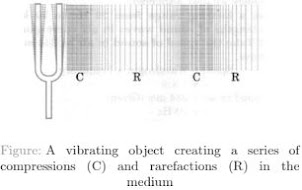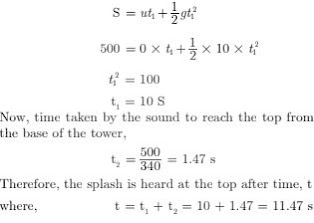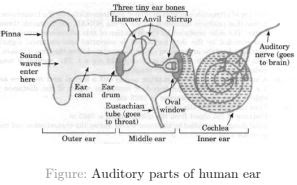# NCERT Solutions for Class 9 Science Chapter 12 Sound

 Chapter Name NCERT Solutions for Class 9 Science Chapter 12 Sound Topics Covered NCERT Intext QuestionsNCERT Exercise Solutions Related Study NCERT Solutions for Class 9 ScienceNCERT Revision Notes for Class 9 ScienceImportant Questions for Class 9 ScienceMCQ for Class 9 ScienceNCERT Exemplar Questions For Class 9 Science

### NCERT Intext Questions

1. How does the sound produced by a vibrating object in a medium reach your ear?

Solution

An object sets the particles of the medium around it vibrating during vibration. The particles in the medium in contact with the vibrating object displace from its equilibrium position. It then exerts force on the adjacent particles. After displacing the adjacent particle, the first particle of medium comes back in its original position. This process continues in the medium till the sound reaches your ear.

2. Explain how sound is produced by your school bell.

Solution

It creates a series of compressions and rarefactions making production of sound, when the bell continues to move forward and backward.

3. Why are sound waves called mechanical waves?

Solution

Sound waves need material medium to propagate. Sound waves propagate through a medium because of the interaction of the particles present in that medium.

4. Suppose you and your friend are on the Moon. Will you be able to hear any sound produced by your friend?

Solution

No, I will not be able to hear any sound produced by my friend because sound waves need a medium through which they can propagate. Since there is no material medium on the Moon due to absence of atmosphere, therefore, I cannot hear any sound on the Moon.

5. Which wave property determines (a) loudness, (b) pitch?

Solution

(a) Amplitude
(b) Frequency

6. Guess which sound has a higher pitch : guitar or car horn?

Solution

High the frequency higher is the pitch. So, guitar has a higher pitch than car horn, because sound produced by the strings of guitar has high frequency than that of car horn.

7. What is wavelength, frequency, time period and amplitude of a sound wave?

Solution

• Wavelength : The distance between two consecutive compressions or two consecutive rarefactions is known as the wavelength. Its SI unit is metre (m).
• Frequency : The number of complete oscillations per unit time is known as the frequency of a sound wave. It is measured in hertz (Hz).
• Amplitude : The maximum height reached by the crest or trough of a sound wave is called its amplitude.

8. How are the wavelength and frequency of a sound wave related to its speed?

Solution

Speed, wavelength and frequency of a sound wave are related by the following equation
Speed (v) = Wavelength (λ) × Frequency (ν)
v = λ × ν

9. Calculate the wavelength of a sound wave whose frequency is 220 Hz and speed is 440 m/s in a given medium.

Solution

Frequency of the sound wave, ν = 220 Hz
Speed of the sound wave, v = 440 ms-1
For a sound wave,
Speed = Wavelength × Frequency;
v = λ × ν
λ = ν /v = 440/220 = 2 m
Hence, the wavelength of the sound wave is 2 m.

10. A person is listening to a tone of 500 Hz sitting at a distance of 450 m from the source of the sound. What is the time interval between successive compressions from the source?

Solution

The time interval between two successive compressions is equal to the time period of the wave. This time period is reciprocal of the frequency of the wave and is given by the relation :
T = 1/Frequency = 1/500 = 0.002 s

11. Distinguish between loudness and intensity of sound.

Solution

Intensity of a sound wave is defined as the amount of sound energy passing through a unit area per second. Loudness is a measure of the response of the ear to the sound. The loudness of a sound is defined by its amplitude. The amplitude of a sound decides its intensity, which in turn is perceived by the ear as loudness.

12. In which of the three media; air, water or iron, does sound travel the fastest at a particular temperature?

Solution

The speed of sound depends on the nature of the medium. Sound travels fastest in solids. Its speed decreases in liquids and it is the slowest in gases. Therefore, for a given temperature, sound travels fastest in iron.

13. An echo returned in 3 s. What is the distance of the reflecting surface from the source, given that the speed of sound is 342 m s–1?

Solution

Speed of sound, v = 342 ms–1
Echo returns in time, t = 3 s
Distance travelled by sound = v × t = 342 × 3 = 1026 m
In the given time interval, sound has to travel a distance that is twice the distance of the reflecting surface and the source.
Hence, the distance of the reflecting surface from the
source = (1026/2)m = 513 m.

14. Why are the ceilings of concert halls curved?

Solution

Ceilings of concert halls are curved so that sound after reflection (from the walls) spreads uniformly in all directions.

15. What is the audible range of the average human ear?

Solution

The audible range of an average human ear lies between 20 Hz to 20,000 Hz.

16. What is the range of frequencies associated with :
(a) Infrasound
(b) Ultrasound

Solution

(a) Infrasound has frequencies less than 20 Hz.
(b) Ultrasound has frequencies more than 20,000 Hz.

17. A submarine emits a sonar pulse, which returns from an underwater cliff in 1.02 s. If the speed of sound in salt water is 1531 m/s, how far away is the cliff from the submarine?

Solution

Time taken by the sonar pulse to return, t = 1.02 s
Speed of sound in salt water, v = 1531 ms–1
Distance of the cliff from the submarine = Speed of sound × Time taken
Distance of the cliff from the submarine = 1.02 × 1531 = 1561.62 m
Distance travelled by the sonar pulse during its transmission and reception in water = 2 × Actual
distance = 2d
Actual Distance,
d = Distance of the cliff from the submarine/2
= 1561.62/2 = 780.81 m

### NCERT Exercise Solutions

1. What is sound and how is it produced?

Solution

Sound is a form of energy which gives the sensation of hearing. It is produced by the vibrations caused in air by vibrating objects.

2. Describe with the help of a diagram, how compressions and rarefactions are produced in air near a source of sound?

Solution

When a vibrating body moves forward, it creates a region of high pressure in its vicinity. This region of high pressure is known as compressions. It creates a region of low pressure in its vicinity when it moves backward. This region is known as a rarefaction. As the body continues to move forward and backwards, it produces a series of compressions and rarefactions, as shown in below figure.3. Give an experiment to show that sound needs a material medium for its propagation.

Solution

Take an electronic bell and an air tight glass bell jar connected to a vacuum pump. Suspend the bell inside the jar and press the switch of the bell. You will be able to hear the bell ring. Now, pump out the air from the glass jar. The sound of the bell will become fainter and after some time, the sound will not be heard. This is so because almost all air has been pumped out. This shows that sound needs a material medium to travel.4. Why is sound wave also called a longitudinal wave?

Solution

The air particle vibrates parallel to the direction of propagation as sound wave is produced by compressions and rarefactions in the air that is why it is called longitudinal wave.

5. Which characteristics of the sound help you to identify your friend by his voice while sitting with others in a dark room?

Solution

The quality or timber of sound enables us to identify our friend by his voice.

6. Flash and thunder are produced simultaneously. But thunder is heard a few seconds after the flash is seen, why?

Solution

The speed of sound (344 m/s) is less than the speed of light (3 × 108 m/s). Sound of thunder takes more time to reach the Earth as compared to light. Hence, a flash is seen before we hear a thunder.

7. A person has a hearing range from 20 Hz to 20 kHz. What are the typical wavelengths of sound waves in air corresponding to these two frequencies? Take the speed of sound in air as 344 m s–1.

Solution

For a sound wave, Speed = Wavelength × Frequency
v = λ × ν
Speed of sound in air = 344 m/s (Given)
(i) For, ν = 20 Hz, λ1 = v/ ν = 344/20 = 17.2 m
(ii) For ν = 20000 Hz, λ2 = v/ ν = 344/20000 = 0.0172 m
Hence, for humans, the wavelength range for hearing is 0.0172 m to 17.2 m.

8. Two children are at opposite ends of an aluminium rod. One strikes the end of the rod with a stone. Find the ratio of times taken by the sound wave in air and in aluminium to reach the second child.

Solution

Velocity of sound in air = 346 m/s
Velocity of sound wave in aluminium = 6420 m/s
Let length of rode be 1 m. Time taken for sound wave
in air,
t1 = 1/Velocity in air
Time taken for sound wave in aluminium,
t2 = 1/Velocity in aluminium
Therefore, t1/t2 = Velocity in aluminium/Velocity in air
= 6420/346 = 18.55

9. The frequency of a source of sound is 100 Hz. How many times does it vibrate in a minute?

Solution

Frequency = 100 Hz (given)
This means the source of sound vibrates 100 times in one second. Therefore, number of vibrations in 1 minute, i.e., in 60 seconds = 100 × 60 = 6000 times

10. Does sound follow the same laws of reflection as light does? Explain.

Solution

Sound follows the same laws of reflection as light does. The incident sound wave and the reflected sound wave make the same angle with the normal to the surface at the point of incidence. Also, the incident sound wave, the reflected sound wave and the normal to the point of incidence all lie in the same plane.

11. When a sound is reflected from a distant object, an echo is produced. Let the distance between the reflecting surface and the source of sound production remains the same. Do you hear echo sound on a hotter day?

Solution

An echo is heard when the time for the reflected sound is heard after 0.1 s
Time taken = Total Distance/Velocity
On a hotter day the velocity of sound is more. If the time taken by echo is less than 0.1 sec it will not be heard.

12. Give two practical applications of reflection of sound waves.

Solution

Two practical applications of reflection of sound waves are :

1. Reflection of sound is used to measure the distance and speed of underwater objects. This method is known as SONAR.
2. Working of a stethoscope is also based on reflection of sound. In a stethoscope, the sound of the patient’s heartbeat reaches the doctor’s ear by multiple reflection of sound.

13. A stone is dropped from the top of a tower 500 m high into a pond of water at the base of the tower. When is the splash heard at the top? Given, g = 10 m s–2 and speed of sound = 340 m s–1.

Solution

Height of the tower, s = 500 m
Velocity of sound, v = 340 m s–1
Acceleration due to gravity, g = 10 m s–2
Initial velocity of the stone, ν = 0 (since the stone is initially at rest)
Time taken by the stone to fall to the base of the tower, t1
According to the second equation of motion :14. A sound wave travels at a speed of 339 m s–1. If its wavelength is 1.5 cm, what is the frequency of the wave? Will it be audible?

Solution

Speed of sound, v = 339 ms-1
Wavelength of sound, λ = 1.5 cm = 0.015 m
Speed of sound = Wavelength × Frequency
v = λ × ν
ν = v/λ = 339/0.015 = 22600 Hz
The frequency range of audible sound for humans lies between 20 Hz to 20,000 Hz. Since, the frequency of the given sound is more than 20,000 Hz, it is not audible.

15. What is reverberation ? How can it be reduced ?

Solution

The repeated multiple reflections of sound in any big enclosed space is known as reverberation.
The reverberation can be reduced by covering th ceiling and walls of the enclosed space with sound absorbing materials, such as fibre board, loose woolens, etc.

16. What is loudness of sound ? What factors does it depend on ?

Solution

The effect produced in the brain by the sound of different frequencies is called loudness of sound. Loudness depends on the amplitude of vibrations. In fact, loudness is proportional to the square of the amplitude of vibrations.

17. Explain, how bats use ultrasound to catch a prey ?

Solution

Bats produce high – pitched ultrasonic squeaks. These high –pitched squeaks are reflectedby objects such as preys and returned to the bat’s ear. This allows a bat to know the distance of his prey.

18. How is ultrasound used for cleaning ?

Solution

Objects to be cleaned are put in a cleaning solution and ultrasonic sound waves are passed through that solution. The high frequency of these ultrasound waves detaches the dirt from the objects.

19. Explain the working and application of a sonar.

Solution

SONAR is an acronym for Sound Navigation And Ranging. It is an acoustic device used to measure the depth, direction, and speed of underwater objects such as submarines and ship wrecks with the help of ultrasounds. It is also used to measure the depth of seas and oceans.

A beam of ultrasonic sound is produced and transmitted by the transducer (it is a device that produces ultrasonic sound) of the SONAR, which travels through sea water. The echo produced by the reflection of this ultrasonic sound is detected and recorded by the detector, which is converted into electrical signals. The distance (d) of the underwater object is calculated from the time (t) taken by the echo to return with speed (v ) is given by 2d = v × t.
This method of measuring distance is also known as ‘echo-ranging’.

20. A sonar device on a submarine sends out a signal and receives an echo 5 s later. Calculate the speed of sound in water if the distance of the object from the submarine is 3625 m.

Solution

Time taken to hear the echo, t = 5s
Distance of the object from the submarine,
d = 3625 m
Total distance travelled by the sonar waves during th transmission and reception in water = 2d
Velocity of sound in water,
v = 2d/t = (2 × 3625)/5 = 1450 ms-1.

21. Explain, how defects in a metal block can be detected using ultrasound ?

Solution

Defects in metal blocks do not allow ultrasound to pass through them and they are reflected back. This fact is used to detect defects in metal blocks. Ultrasound is passed through one end of a metal block and detectors are placed on the other end. The defective part of the metal block does not allow ultrasound to pass through it. As a result, it will not be detected by the detector.
Hence, defects in metal blocks can be detected using ultrasound.22. Explain, how the human ear works ?

Solution

The human ear consists of three parts - the outer ear, middle ear and inner ear.

1. Outer ear: This is also called ‘pinna’. It collects the sound from the surrounding and directs it towards auditory canal.
2. Middle ear: The sound reaches the end of the auditory canal where there is a thin membrane called eardrum or tympanic membrane. The sound waves set this membrane to vibrate. These vibrations are amplified by three small bones - hammer, anvil and stirrup.
3. Inner ear: These vibration reach the cochlea in the inner ear and are converted into electrical signals which are sent to the brain by the auditory nerve, and the brain interprets them as sound.